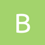# How Cow!

Limits: 1s, 512 MB

There is a cow in a village. She can eat as much grasses as she want. Her desire is to grab grass is up to infinity. This is a matter of ‘LOL’ that, there is a field with infinite circumstances and the field was full of green and tasty grasses.

This is a companion discussion topic for the original entry at https://toph.co/p/how-cow

My calculator says: 578 x 578 x pi = 1049555.84

In this problem they taken the value of pi is 3.1416

1 Like
``````#include<stdio.h>
int main()
{
int n,i;
scanf("%d",&n);
int a[n];
for(i=0;i<n;i++){
scanf("%d",&a[i]);
}
float c=acos(-1);

float b[n];
for(i=0;i<n;i++){
b[i]=c*(float)a[i]*(float)a[i];

}

for(i=0;i<n;i++){
printf("%.3f\n",b[i]);
}
return 0;

}
``````

why is it showing wrong ans?

I got AC for the first bunch of input . but what’s wrong with second one?
my code

``````    for(var i=1;i<c.length;i++)
{
var temp = c[i]*c[i]*3.1416+""
console.log((temp.slice(0,temp.indexOf('.')+4)))
}``````

The reason is already pointed out by @bisnu_sarkarbisnu_sarkar

Feb 26

In this problem they taken the value of pi is 3.1416

``````#include <stdio.h>

int main() {

float area;
int T,L;
scanf("%d",&T);

while(T--){

scanf("%d",&L);
float pie;
pie=3.1416;
area = pie * L * L;

printf("%.3f",area);

}
return 0;
}
``````

what is the problem??

You seem to have forgooten to print newline (’\n’)

pi=3.1416 diye korlei ac hbe
kintu acos(-1) dile wa

``````#include<bits/stdc++.h>
#include <iomanip>
#include<iostream>

#define PI 3.1416

using namespace std;

int main(){
int t;
cin >> t;
float n[t], area[t];
for(int i=0; i<t; i++){
cin >> n[i];
}

for(int i=0; i<t; i++){
area[i] = PI * n[i] * n[i];
}

for(int i=0; i<t; i++){
cout << fixed << showpoint;
cout << setprecision(3);
cout << area[i] << endl;
}
return 0;
}
```what is wrong in my code ?

Try taking double instead of float.

``````#include<bits/stdc++.h>
using namespace std;
#define ll long long int
#define max_3(a,b,c)    max(a, max(b,c))
#define pi              2*acos(0.0)
int main()
{
float t,n,c=3.1415991188,d;
cin>>t;
while(t--){
cin>>n;
d=c*pow(n,2);
printf("%.3f\n",d);
}
}
``````

but its show wrong ans

T=int(input())
for x in range(1,T+1):
L=int(input())
a=3.1416LL
print(round(a,3))